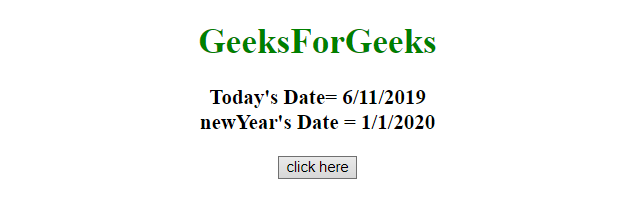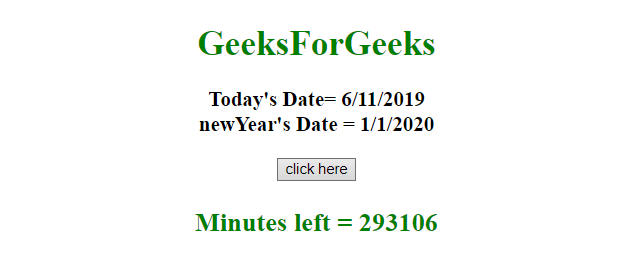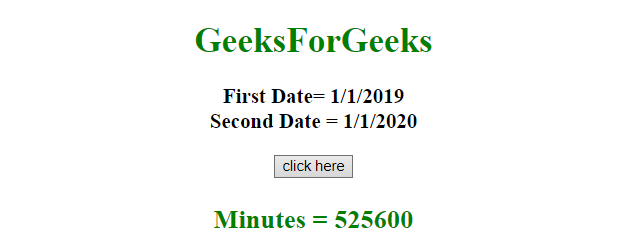# How to calculate minutes between two dates in JavaScript ?

Given two dates and the task is to get the number of minutes between them using JavaScript.

Approach:

• Initialize both Date object.
• Subtract older date from the new date. It will give the number of milliseconds from 1 January, 1970.
• Convert milliseconds to minutes .

Example 1: This example uses the current date and next newYear date to get the difference of dates in minutes.

 `  ` `<``html``>  ` `    ``<``head``>  ` `        ``<``title``>  ` `            ``How to get the minutes between ` `            ``two dates in JavaScript ? ` `        `` ` `    ``  ` `     `  `    ``<``body` `style` `= ``"text-align:center;"``>  ` `         `  `        ``<``h1` `style` `= ``"color:green;"` `>  ` `            ``GeeksForGeeks  ` `        `` ` `         `  `        ``<``p` `id` `= ``"GFG_UP"` `style` `=  ` `            ``"font-size: 19px; font-weight: bold;"``> ` `        `` ` `         `  `        ``<``button` `onClick` `= ``"GFG_Fun()"``> ` `            ``click here ` `        `` ` `         `  `        ``<``p` `id` `= ``"GFG_DOWN"` `style` `=  ` `            ``"color: green; font-size: 24px; font-weight: bold;"``> ` `        `` ` `         `  `        ``` `        ``<``script``> ` `            ``var up = document.getElementById('GFG_UP'); ` `            ``var down = document.getElementById('GFG_DOWN'); ` `             `  `            ``// Declare dates ` `            ``var today = new Date(); ` `            ``var newYear = new Date("01-01-2020"); ` `             `  `            ``// Display the dates ` `            ``up.innerHTML = "Today's Date= "+ today.toLocaleDateString() ` `                ``+ "<``br``> newYear's Date = " + newYear.toLocaleDateString(); ` `             `  `            ``// Function to calculate difference ` `            ``// between two dates ` `            ``function GFG_Fun() { ` `                ``var dif = (newYear - today); ` `                ``var dif = Math.round((dif/1000)/60); ` `                ``down.innerHTML = "Minutes left = " + dif; ` `            ``} ` `        ``  ` `    ``  ` ` `

Output:

• Before clicking on the button:• After clicking on the button:Example 2: This example uses the 2019 newYear and 2020 newYear date to get the difference in minutes.

 `  ` `<``html``>  ` `    ``<``head``>  ` `        ``<``title``>  ` `            ``How to get the minutes between ` `            ``two dates in JavaScript ? ` `        `` ` `    ``  ` `     `  `    ``<``body` `style` `= ``"text-align:center;"``>  ` `         `  `        ``<``h1` `style` `= ``"color:green;"` `>  ` `            ``GeeksForGeeks  ` `        `` ` `         `  `        ``<``p` `id` `= ``"GFG_UP"` `style` `=  ` `            ``"font-size: 19px; font-weight: bold;"``> ` `        `` ` `         `  `        ``<``button` `onClick` `= ``"GFG_Fun()"``> ` `            ``click here ` `        `` ` `         `  `        ``<``p` `id` `= ``"GFG_DOWN"` `style` `=  ` `            ``"color: green; font-size: 24px; font-weight: bold;"``> ` `        `` ` `         `  `        ``` `        ``<``script``> ` `            ``var up = document.getElementById('GFG_UP'); ` `            ``var down = document.getElementById('GFG_DOWN'); ` `             `  `            ``// Declare dates ` `            ``var newYear1 = new Date("01-01-2019"); ` `            ``var newYear2 = new Date("01-01-2020"); ` `             `  `            ``// Display the dates ` `            ``up.innerHTML = "First Date= "+ newYear1.toLocaleDateString() ` `                ``+ "<``br``> Second Date = " + newYear2.toLocaleDateString(); ` `             `  `            ``// Function to calculate difference ` `            ``// between two dates ` `            ``function GFG_Fun() { ` `                ``var dif = (newYear2 - newYear1); ` `                ``var dif = Math.round((dif/1000)/60); ` `                ``down.innerHTML = "Minutes left = " + dif; ` `            ``} ` `        ``  ` `    ``  ` `                     `

Output:

• Before clicking on the button:• After clicking on the button:My Personal Notes arrow_drop_upCheck out this Author's contributed articles.

If you like GeeksforGeeks and would like to contribute, you can also write an article using contribute.geeksforgeeks.org or mail your article to contribute@geeksforgeeks.org. See your article appearing on the GeeksforGeeks main page and help other Geeks.

Please Improve this article if you find anything incorrect by clicking on the "Improve Article" button below.